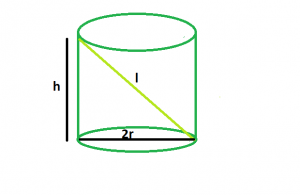# Longest rod that can be inserted within a right circular cylinder

Given a right circular cylinder of height, & radius. The task is to find the length of the longest rod that can be inserted within it.

Examples:

```Input : h = 4, r = 1.5
Output : 5

Input : h= 12, r = 2.5
Output : 13
```

## Recommended: Please try your approach on {IDE} first, before moving on to the solution.Approach:
From the figure, it is clear that we can get the length of the rod by using pythagoras theorem, by treating the height of cylinder as perpendicular, diameter as base and length of rod as hypotenuse.

So, l2 = h2 + 4*r2.

Therefore,

```l = √(h2 + 4*r2)
```

Below is the implementation of the above approach:

## C++

 `// C++ Program to find the longest rod ` `// that can be fit within a right circular cylinder ` `#include ` `using` `namespace` `std; ` ` `  `// Function to find the side of the cube ` `float` `rod(``float` `h, ``float` `r) ` `{ ` ` `  `    ``// height and radius cannot be negative ` `    ``if` `(h < 0 && r < 0) ` `        ``return` `-1; ` ` `  `    ``// length of rod ` `    ``float` `l = ``sqrt``(``pow``(h, 2) + 4 * ``pow``(r, 2)); ` `    ``return` `l; ` `} ` ` `  `// Driver code ` `int` `main() ` `{ ` `    ``float` `h = 4, r = 1.5; ` ` `  `    ``cout << rod(h, r) << endl; ` ` `  `    ``return` `0; ` `} `

## Java

 `// Java Program to find the longest rod ` `// that can be fit within a right circular cylinder ` ` `  `import` `java.io.*; ` ` `  `class` `GFG { ` `    `  ` `  `// Function to find the side of the cube ` `static` `float` `rod(``float` `h, ``float` `r) ` `{ ` ` `  `    ``// height and radius cannot be negative ` `    ``if` `(h < ``0` `&& r < ``0``) ` `        ``return` `-``1``; ` ` `  `    ``// length of rod ` `    ``float` `l = (``float``)(Math.sqrt(Math.pow(h, ``2``) + ``4` `* Math.pow(r, ``2``))); ` `    ``return` `l; ` `} ` ` `  `// Driver code ` ` `  ` `  `    ``public` `static` `void` `main (String[] args) { ` `            ``float` `h = ``4``;  ` `            ``float` `r = ``1``.5f; ` `            ``System.out.print(rod(h, r)); ` `    ``} ` `} ` `// This code is contributed by anuj_67.. `

## Python 3

 `# Python 3 Program to find the longest  ` `# rod that can be fit within a right  ` `# circular cylinder ` `import` `math  ` ` `  `# Function to find the side of the cube ` `def` `rod(h, r): ` `     `  `    ``# height and radius cannot  ` `    ``# be negative ` `    ``if` `(h < ``0` `and` `r < ``0``): ` `        ``return` `-``1` ` `  `    ``# length of rod ` `    ``l ``=` `(math.sqrt(math.``pow``(h, ``2``) ``+`  `               ``4` `*` `math.``pow``(r, ``2``))) ` `    ``return` `float``(l) ` ` `  `# Driver code ` `h , r ``=` `4``, ``1.5` `print``(rod(h, r)) ` ` `  `# This code is contributed ` `# by PrinciRaj1992 `

## C#

 `// C# Program to find the longest  ` `// rod that can be fit within a  ` `// right circular cylinder ` `using` `System; ` ` `  `class` `GFG ` `{ ` ` `  `// Function to find the side ` `// of the cube ` `static` `float` `rod(``float` `h, ``float` `r) ` `{ ` ` `  `    ``// height and radius cannot  ` `    ``// be negative ` `    ``if` `(h < 0 && r < 0) ` `        ``return` `-1; ` ` `  `    ``// length of rod ` `    ``float` `l = (``float``)(Math.Sqrt(Math.Pow(h, 2) +  ` `                            ``4 * Math.Pow(r, 2))); ` `    ``return` `l; ` `} ` ` `  `// Driver code ` `public` `static` `void` `Main () ` `{ ` `    ``float` `h = 4;  ` `    ``float` `r = 1.5f; ` `    ``Console.WriteLine(rod(h, r)); ` `} ` `} ` ` `  `// This code is contributed by shs `

## PHP

 ` `

Output:

```5
```

Attention reader! Don’t stop learning now. Get hold of all the important DSA concepts with the DSA Self Paced Course at a student-friendly price and become industry ready.

My Personal Notes arrow_drop_upCheck out this Author's contributed articles.

If you like GeeksforGeeks and would like to contribute, you can also write an article using contribute.geeksforgeeks.org or mail your article to contribute@geeksforgeeks.org. See your article appearing on the GeeksforGeeks main page and help other Geeks.

Please Improve this article if you find anything incorrect by clicking on the "Improve Article" button below.